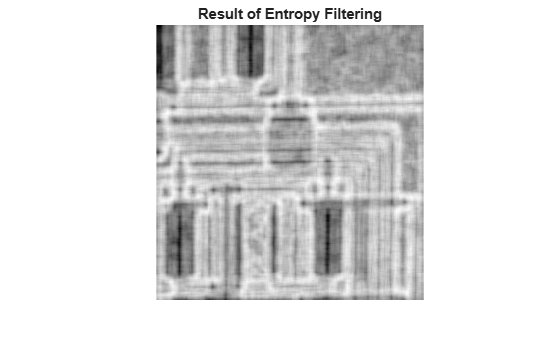# entropyfilt

Local entropy of grayscale image

## Syntax

``J = entropyfilt(I)``
``J = entropyfilt(I,nhood)``

## Description

example

````J = entropyfilt(I)` returns the array `J`, where each output pixel contains the entropy value of the 9-by-9 neighborhood around the corresponding pixel in the input image `I`. For pixels on the borders of `I`, `entropyfilt` uses symmetric padding. In symmetric padding, the values of padding pixels are a mirror reflection of the border pixels in `I`.```
````J = entropyfilt(I,nhood)` performs entropy filtering of the input image `I` using the neighborhood `nhood`.```

## Examples

collapse all

This example shows how to perform entropy filtering using `entropyfilt`. Brighter pixels in the filtered image correspond to neighborhoods in the original image with higher entropy.

Read an image into the workspace.

`I = imread('circuit.tif');`

Perform entropy filtering using `entropyfilt`.

`J = entropyfilt(I);`

Show the original image and the processed image.

```imshow(I) title('Original Image')``````figure imshow(J,[]) title('Result of Entropy Filtering')```## Input Arguments

collapse all

Image to be filtered, specified as a numeric array of any dimension. If the input image has more than two dimensions (```ndims(I) > 2```), such as for an RGB image, then `entropyfilt` filters all 2-D planes along the higher dimensions. For data types `double` and `single`, the intensity values of `I` must be in the range [0, 1].

Data Types: `double` | `uint8` | `uint16` | `uint32` | `logical`

Neighborhood, specified as a numeric or logical array containing `0`s and `1`s. The size of `nhood` must be odd in each dimension.

By default, `entropyfilt` uses the neighborhood `true(9)`. The center element of the neighborhood is `floor((size(nhood) + 1)/2)`.

To specify neighborhoods of other shapes, such as a disk, use the `strel` function to create a structuring element object of the desired shape. Then, extract the neighborhood from the structuring element object’s `neighborhood` property.

Data Types: `single` | `double` | `int8` | `int16` | `int32` | `int64` | `uint8` | `uint16` | `uint32` | `uint64` | `logical`

## Output Arguments

collapse all

Filtered image, returned as a numeric array the same size as the input image `I`. The data type of `J` is `double`. If the input image `I` is a binary image (data type `logical`), then the values of `J` are in the range [0, 1]. For all other data types of `I`, the values of `J` are in the range [0, 8].

Data Types: `double`

collapse all

### Entropy

Entropy is a statistical measure of randomness that can be used to characterize the texture of the input image.

Entropy is defined as `-sum(p.*log2(p))`, where `p` contains the normalized histogram counts returned from `imhist`.

## Tips

• By default, `entropyfilt` uses two bins for logical arrays. `entropyfilt` converts any other class to `uint8` for the histogram count calculation and uses 256 bins so that the pixel values are discrete and directly correspond to a bin value.

• If the input image `I` is a grayscale image, then the values of `J` can exceed the range [0, 1] that some Image Processing Toolbox™ functions expect for images of type `double`. To pass `J` as an input argument to these functions, use the `rescale` function to rescale the values of `J` to the range [0, 1].

 Gonzalez, R. C., R. E. Woods, and S. L. Eddins. Digital Image Processing Using MATLAB. New Jersey, Prentice Hall, 2003, Chapter 11.